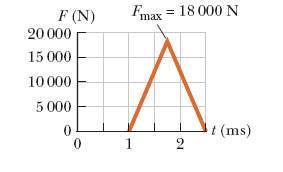# Problem: An estimated force vs. time curve for a baseball struck by a bat is shown in the figure below.(a) From the curve, determine the impulse delivered to the ball.(b) From the curve, determine the average force exerted on the ball.

###### FREE Expert Solution

(a) Impulse:

$\overline{){\mathbf{I}}{\mathbf{=}}{\mathbf{Ft}}}$

Therefore, the impulse is the area under the curve:###### Problem Details

An estimated force vs. time curve for a baseball struck by a bat is shown in the figure below.(a) From the curve, determine the impulse delivered to the ball.

(b) From the curve, determine the average force exerted on the ball.